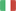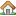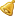EnglishItalianoVisitatore
Torna allaHomepage...

###Generali:

• Dipartimento: Ingegneria
• Tipologia: Corso Di Laurea Dm.270/04
• Corso di Laurea: Ingegneria Gestionale
• Settore Ministeriale: MAT/05
• Codice di verbalizzazione: 8037326
• Metodi di insegnamento: Laboratorio
• Metodi di valutazione: Scritto E Orale
• Prerequisiti: Knowledge of the program of a first year "Analysis 1)" that is 1) compute the limit of a real funcion on one variable 2) to analyze the grafh on a real function of one variable 3) compute the derivatives and integrals a real function of one vriable 4) be able to study the bahavior of a real function of one variable in the neighborhood of a point and in particular how to use the Taylor expansion both with Peano and Lagrange "Rest" 5) be able to compute the 6) numerical series 7) improper integrals of real function of one real variable 8) limit of real functions of more than one variable 9) compute the partial derivatives 10) be able to establish the presence of the tangent plane wich is equivalent to the differentiability of the function.
• Obiettivi: At the end of the course the students are supposed to be able to handle the mathematical tecniques learnt during the course. In particular the are supposed to 1) be able to integrate real functions of more than one variable 2) to be able to compute the line-integrals of the first and second kind (differential forms) 3) to be able to find the extremum points, either constrained or not) of functions of several variables 4) be able to integrate functions of several variable both computing ordinary and improper integrals
• Ricevimento: Su appuntamento da fissarsi tramite posta elettronica

###Didattica:

• A.A.: 2018/2019
• Canale: Canale 1
• Crediti (CFU): 9
• Obbligo di Frequenza: No